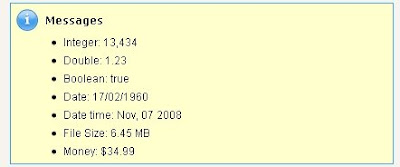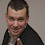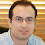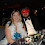### A Utility Apex Class to Convert All Types Into String

If you have been in the programming world long enough, you already know that casting types from one to another specially string representation of the variables is always part the job.

Whether you want to show the data to user or form the variables in different formats, incorporate them in messages, etc.

In this article I will present you one of the Apex classes I have written to help me faster develop Salesforce Applications.

This class helps me in number of ways, for example I do not need to worry about the underlying Apex code to convert a primitive type to string anymore, for all types I just need to call one method "ToString" and it will take care of for me.
Also the methods provide me with formatting capabilities, so I can not only convert to string but also format the string in many ways I need.

Look at the below code and see how the results are:

```ApexPages.addmessage(new ApexPages.message(ApexPages.severity.INFO,'Integer: '+ zConvert.ToString(13434)));
ApexPages.addmessage(new ApexPages.message(ApexPages.severity.INFO,'Date: ' + zConvert.ToString(date.newinstance(1960, 2, 17))));
ApexPages.addmessage(new ApexPages.message(ApexPages.severity.INFO,'Date time: ' + zConvert.ToString(Datetime.now(),'MMM, dd yyyy')));

This is the result of running the above code:Well, very nice, now let's see how the actual class is developed:
Because I would like to directly call my Coverter's class methods without creating a new instance of the class, I have defined all the methods as "static".

The methods for this class are:
• ToString(Integer)
• ToString(Double)
• ToString(Long)
• ToString(Boolean)
• ToString(Date)
• ToString(Date,format)
sample: zConvert.ToString(mydate,'MM-dd-yy')
• ToString(Time)
• ToString(Time,format)
sample: zConvert.ToString(myTime,'hh:mm:ss')
• ToString(Datetime)
• ToString(Datetime,format)
• ToString(Decimal)
• ToString(Decimal, ScientificNotaion)
ScientificNotaion is a Boolean value and if false is passed then the string will not have scientific notations.
• FileSizeToString(Long)
Returns values such as "5.5 KB", "8 MB", etc. Parameter passed is in bytes.
• CurrencyToString(Decimal, CurrencyChar)
CurrencyChar can be "\$", "£", etc

```public class zConvert
{
/* The Initial Developer of the Original Code is Sam Arjmandi.
* Portions created by the Initial Developer are Copyright (C) 2008
*
* This Code is provided "As Is" without warranty of any kind.
*/

public static String ToString(integer Value)
{
/* string representation if an Integer value */
return Value.format();
}

public static String ToString(Double Value)
{
/* string representation if a Double value */
return Value.format();
}

public static String ToString(Boolean Value)
{
/* string representation if a Boolean value */
if (Value)
return 'true';
else
return 'false';
}

public static String ToString(Long Value)
{
/* string representation if a Long value */
return Value.format();
}

public static String ToString(Date Value)
{
/* string representation if a Date value */
return Value.format();
}

public static String ToString(Date Value,String format)
{
/* string representation if a Date value with formatting */
Datetime temp = Datetime.newInstance(Value.year(), Value.month(), Value.day());
return temp.format(format);
}

public static String ToString(Datetime Value)
{
/* string representation if a Datetime value */
return Value.format();
}

public static String ToString(Datetime Value,String format)
{
/* string representation if a Datetime value with formatting */
return Value.format(format);
}

public static String ToString(Time Value)
{
/* string representation if a Time value */
return String.valueOf(Value);
}

public static String ToString(Time Value, String format)
{
/* string representation if a Time value with formating */
Datetime temp = Datetime.newInstance(1970, 1, 1, Value.hour(), Value.minute(), Value.second());
return temp.format(format);
}

public static String ToString(Decimal Value)
{
/* string representation if a Decimal value */
return Value.format();
}

public static String ToString(Decimal Value, Boolean ScientificNotation)
{
/* string representation if a Decimal value with or without Scientific Notation */
if (ScientificNotation)
return Value.format();
else
return Value.toPlainString();
}

public static String FileSizeToString(Long Value)
{
/* string representation if a file's size, such as 2 KB, 4.1 MB, etc */
if (Value < 1024)
else
if (Value >= 1024 && Value < (1024*1024))
{
//KB
Decimal kb = Decimal.valueOf(Value);
kb = kb.divide(1024,2);
}
else
if (Value >= (1024*1024) && Value < (1024*1024*1024))
{
//MB
Decimal mb = Decimal.valueOf(Value);
mb = mb.divide((1024*1024),2);
}
else
{
//GB
Decimal gb = Decimal.valueOf(Value);
gb = gb.divide((1024*1024*1024),2);

}

}

public static String CurrencyToString(Decimal Value, String CurrencyChar)
{
return CurrencyChar + ToString(Value);
}

}```

1.Thanks Sam!

One sort of unrelated question: I still haven't figured out how to make use of the ApexPages.severity enums to display them nicely.
How does your VF code look that displays the nice yellow box and the 7 ToString'ed values in the first part of the post? The documentation, unfortunately, haven't been able to help me (or maybe I've not managed to understand it...).

If you have any input on this subject, I'd appreciate it.

Thanks
//Johan Liljegren

2.Good work. How do you consistently return 2 decimal places for a formatted decimal? For example you have a list of decimal values and you want to render

123,456.78
234,567.80
345,678.00

instead of (what this class does, I believe):

123,456.78
234,567.8
345,678

3.use .format() method
.....Alonso

4.This comment has been removed by a blog administrator.

5.Any idea on how to convert the SalesForce type of ID to string?

6.Mark, You can cast ID type to string implicitly.
Ex: string id = Account.Id;

7.I am trying to create a formula that is calucating a % from a roll-up summary field and a text field that has a currency value. I can not change the text field to a Number field because it is from a appexchange package. How can I convert my formula so it will accept the text field? Text field is SalesVision_c__Total_Asset_Millions__c

IF(SalesVision_c__Total_Assets_Millions__c> 0, SalesVision_AUM__c / (SalesVision_c__Total_Assets_Millions__c * 1000000), 0)

8.Hi Hart,
May you need to cast that value into number data type before using it in the formula you posted above.

9.First of all, very nice work. I am going to be needing to use this very soon (like... today). Thank you very much for posting your work. With that said, when attempting to use this I get the error

Save error: Static methods cannot be invoked through an object instance: ToString(Integer)

I'm not exactly sure what that means. In an apex class, I just put the line

String testVal = zConvert.ToString(13434);

and it threw that. Any ideas?

10.Hey,
You must have created an instance of the zConvert class. You do not need to get an instance. All methods are static and therefore you can use them directly using the class name.

11.Cheers, thanks for the class. Any chance there's a test class for this? Deadlines loom and creating a test is a chore. Either way this is a great help.

12.definitely useful, Sam. Thank you for sharing!

Here's 100% test coverage:

/* test string utils */
system.assertEquals('true',zConvert.ToString(true));
system.assertEquals('false',zConvert.ToString(false));
system.assertEquals('4/17/1960',zConvert.ToString(date.newInstance(1960, 4, 17)));
system.assertEquals('Apr, 26 04 11:24:40',zConvert.ToString(datetime.newInstance(2004, 4, 26, 23, 24, 40), 'MMM, dd yy hh:mm:ss'));
system.assertEquals('Apr, 17 1960',zConvert.ToString(date.newInstance(1960, 4, 17), 'MMM, dd yyyy'));
system.assertEquals('4/26/2004 11:24 PM',zConvert.ToString(datetime.newInstance(2004, 4, 26, 23, 24, 40)));
system.assertEquals('12.457',zConvert.ToString(decimal.valueOf('12.4567')));
system.assertEquals('0',zConvert.ToString(decimal.valueOf('.000000000000000000000012'), true));
system.assertEquals('12.4567',zConvert.ToString(decimal.valueOf('12.4567'), false));
system.assertEquals('3.142',zConvert.ToString(double.valueOf('3.14159')));
system.assertEquals('123,456',zConvert.ToString(123456));
system.assertEquals('1,234,567,890',zConvert.ToString(long.valueOf('1234567890')));
system.assertEquals('18:30:02.020Z',zConvert.ToString(time.newInstance(18, 30, 2, 20)));
system.assertEquals('06-30-02-302',zConvert.ToString(time.newInstance(18, 30, 2, 20), 'hh-mm-ss-ms'));
system.assertEquals('\$123,456.17',zConvert.CurrencyToString(decimal.valueOf('123456.17'), '\$'));
system.assertEquals('1,023 Bytes',zConvert.FileSizeToString(long.valueOf('1023')));
system.assertEquals('1,015.62 KB',zConvert.FileSizeToString(long.valueOf('1040000')));
system.assertEquals('1,020.43 MB',zConvert.FileSizeToString(long.valueOf('1070000000')));
system.assertEquals('1,015.14 GB',zConvert.FileSizeToString(long.valueOf('1090000000000')));

13.Hi Sam Arjmandi this is suman your code is good . i faced one problem using apex class and page inserted records from csv file into custom object in salesforce. i take 3 fields in object. one field data type is currency. but uploading time showing error i.e. "llegal assignment from String to Decimal" . i use your code converting string to decimal but that time also showing error what is the solution please let me know

14.Hi Sam

I need to convert Blob to String.Blob is the attachment Body and I need to convert it to String to display it on the VF Page.Can you please suggest any solution for this.

15.Hi Sam,

How do you convert Email Type to string? I am tring to send an email to the current user. I can use User.Email; however, it is of type Email and I cannot get the string value from it.

16.Email, Phone, etc are logical types and have nothing to do with Apex.

In Apex, Email, Phone, etc all of them are string.

17.Hi Sam,

Is there any way we can convert a sObject to String type.

Thanks

18.Hi Sam Arjmandi,
Nice post.In my requirement Object like below form
string strtemp = 'Mahesh__'+strFieldName + '__c';

How to use this String as like object ?(after only i will give these related fields edit and del functionalities)